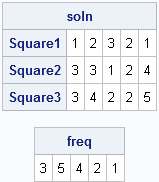I previously wrote about an intriguing math puzzle that involves 5-digit numbers with certain properties. This post presents my solution in the SAS/IML language.

It is easy to generate all 5-digit perfect squares, but the remainder of the problem involves looking at the digits of the squares. For this reason, I converted the set of all 5-digit numbers into an n x 5 array. I used the PUTN function to convert the numbers to strings, and then used the SUBSTR function to extract the first, second, third, fourth, and fifth digits into columns. (I then used the NUM function to change the character array back into a numeric matrix, but this is not necessary.)

The solution enables me to highlight three new functions in SAS 9.3:
• The ELEMENT function enables you to find which elements in one set are contained in another set. I use this function to get rid of all 5-digit perfect squares that contain the digits {6,7,8,9,0}.
• The ALLCOMB function generates all combinations of n elements taken k at a time. After I reduced the problem to a set of nine 5-digit numbers, I used the ALLCOMB function to look at all triplets of the candidate numbers.
• The TABULATE subroutine computes the frequency distribution of elements in a vector or matrix. I used this subroutine to check the frequency of the digits in the triplets of numbers.

Here is my commented solution:

```proc iml; /* generate all 5-digit squares */ f0 = ceil(sqrt(10000)); f1 = floor(sqrt(99999)); AllSquares = T(f0:f1)##2;   /* convert to (n x 5) character array */ c = putn(AllSquares,"5.0"); m = j(nrow(c), 5," "); do i = 1 to 5; m[,i] = substr(c,i,1); end; m = num(m); /* convert to (n x 5) numerical matrix */   /* The numbers are clearly 1,2,3,4,5, since there are 15 digits and each appears a unique number of times. Get rid of any rows that don't have these digits. */ bad = (6:9) || 0; b = element(m, bad); /* ELEMENT: SAS/IML 9.3 function */ hasBad = b[ ,+]; /* sum across columns */ g = m[loc(hasBad=0),]; /* only nine perfect squares left! */   /* Look at all 3-way combinations */ k = allcomb(nrow(g),3);/* ALLCOMB: SAS/IML 9.3 function */ SolnNumber=0; /* how many solutions found? */ do i = 1 to nrow(k); soln = g[k[i,], ]; /* 3x5 matrix */ /* The frequencies of the digits should be 1,2,3,4,5 and the freq of a digit cannot equal the digit */ call tabulate(levels, freq, soln); /* /* TABULATE: SAS/IML 9.3 function */ if ncol(unique(freq))=5 then do; /* are there five freqs? */ if ^any(freq=(1:5)) then do; /* any freq same as digit? */ SolnNumber = SolnNumber+1; print "********" SolnNumber "********", soln[r={"Square1" "Square2" "Square3"}], freq; end; end; end;```

At first, I didn't understand the last clue, so I printed out all seven triplets of numbers that satisfied the first five conditions. When I looked at the output, I finally made sense of the last clue, which is "If you knew which digit I have used just once, you could deduce my three squares with certainty." This told me to look closely at the FREQ vectors in the output. Of the seven solutions, one has frequency vector {3 5 4 2 1}, which means that the 1 digit appears three times, the 2 digit appears five times, and so on to the 5 digit, which appears once. In all of the other solutions, it is the 3 digit that appears once. Therefore, there is a unique solution in which the 5 digit appears only one time. The solution is as follows:The SAS/IML language gave me some powerful tools that I used to solve the math puzzle. I'm particularly pleased that I only used two loops to solve this problem. I was able to vectorize the other computations.

Can you improve my solution? Use the comment to post (or link to) your program that solves the problem. The original post on the SAS Discussion Forum includes other ways to solve the problem in SAS.

ShareDistinguished Researcher in Computational Statistics

Rick Wicklin, PhD, is a distinguished researcher in computational statistics at SAS and is a principal developer of SAS/IML software. His areas of expertise include computational statistics, simulation, statistical graphics, and modern methods in statistical data analysis. Rick is author of the books Statistical Programming with SAS/IML Software and Simulating Data with SAS.

1.Someone asked how long the program takes to find the solution. It's essentially instantaneous. If you delete the PRINT statement, it takes 0.01-0.04 seconds, so that's the "computational time." As published (printing to the default HTML destination), it takes about 0.4 seconds to run, write the output, and update the HTML Results Viewer.

•6 11 25
8 6 16
12 5 ?
i want the solution of this puzzle

•(2*middle num)+half of the first num = 3rd column
(2*11)+3=25
(2*6)+4=16
(2*5)+6=16

2.Hello satish , could u gimme the solution .. cant find the answer.. did u find it

•brother i too dunt have a solid explanation but here it comes
2X3 11X1 5X5
2X4 2X3 2X8
2X6 5X1 ?
what im doing here is factorising the elements in a way that i only choose the smallest prime number (other than one ) now in first row (3+1) +1 =5 and in the same manner 4+3+1=8 and in the last 6+1+1=8 therefore 2X8 =16 (since i was given 10 ,12,14,16)as options and all have 2 as the least valued prime no

•6 =2X3 11 25 2×11+3=25
8 =2X4 6 16 2×6+4=16
12 =2X6 5 ? 2×5+6=16

? = 16
Is this solution ok?

3.8 11 25
6 6 16
? 5 16
i want the solution of this puzzle

•i think may be question is
6 11 25
8 6 16
12 5 16